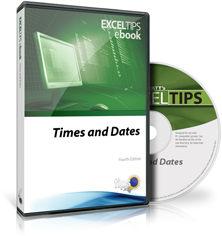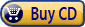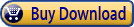Summary: Excel is great at storing all types of data, including times and dates. ExcelTips: Times and Dates provides the details you need to effectively work with this type of data; this is the complete table of contents.ExcelTips: Times and Dates includes 280 information-packed pages that will help you learn exactly how to work with dates and times in Excel. The following is the Table of Contents for the eighth edition:

Introduction

• A Word about Versions
• Date and Time Functions
• Need More Tips?
• A Special Note of Thanks
• Sharing this E-Book

General Date and Time Information

• How Excel Stores Dates and Times
• Changing Excel's Starting Date
• Inserting the Date and Time
• Entering Dates in Excel
• Quickly Entering Dates and Times
• Inserting the Current Time with Seconds
• Dates Copied Incorrectly
• Using Early Dates
• AutoFilling with Weekdays
• Using an Input Mask
• Displaying an Input Format in a Cell
• Defeating Automatic Date Parsing
• Inserting Tomorrow's Date
• Can't Enter Years in a Cell
• Entering the Current Time
• Shortcut to Enter GMT
• Entering Negative Times
• Automatically Entering a Data Entry Time
• Checking for Data Entry Errors for Times
• Converting Dates to Text
• Converting Text to Values
• Backwards Date Parsing
• Defeating Date Parsing when Pasting Information
• Stopping Date Parsing when Opening a CSV File

Worksheet Functions Related to Times and Dates

• Using the EOMONTH Function
• EOMONTH Function is Flakey
• Using the WEEKNUM Function
• The EDATE Function
• Checking for Time Input
• Calculating Fractions of Years
• Calculating Months of Tenure
• Specifying Different Weekends with NETWORKDAYS
• Parsing Non-Standard Date Formats
• Setting a Default Date Format

Time Calculations

• Dealing with Small Time Values
• Entering Large Time Values
• Dealing with Large Numbers of Seconds
• Counting Times within a Range
• Working with Elapsed Time
• Calculating Elapsed Time with Excluded Periods
• Rounding Time
• Rounding to the Nearest Quarter Hour
• Adjusting Times for Time Zones
• Taking the Time into Account in a Formula
• Dealing with Midnight Ending a Day
• Calculating Time Differences between Two Machines
• Calculating TV Time

Date Calculations

• Calculating Averages by Date
• Calculating Future Workdays
• Finding the Previous Work Day
• Calculating Week-Ending Dates
• Calculating Weekend Dates
• Calculating the Last Day in a Week Number
• Using a Week Number as One Criterion in a Formula
• End-of-Month Calculations
• Calculating the First Business Day of the Month
• Calculating a Date Five Days before the First Business Day
• Determining If a Year is a Leap Year
• Leap Years and Fiscal Periods
• Calculating Business Days
• Calculating Months for Billing Purposes
• Determining Business Quarters from Dates
• The Last Business Day
• Forcing Dates Forward
• Pushing Dates Into Last Month
• Calculating the Day of the Year
• Days Left in the Year
• Counting Dates in a Range
• Determining Month Names for a Range of Dates
• Weekdays in a Month
• Counting Month Ends
• Monthly Close-Out Dates
• Listing Dates at Regular Intervals
• Date for Next Wednesday
• Incrementing Months in Dates
• Copying Dates a Year Into the Future
• Noting Inactivity within a Timeframe
• Counting Jobs Completed On a Date
• Determining If a Date is between Other Dates
• Finding the Dates for Minimums and Maximums
• Returning Nothing If Two Values are Empty
• Returning a Weight and a Date
• Determining a Name for a Week Number
• Finding the Date Associated with a Negative Value
• Averaging Values for a Given Month and Year
• Filtering for Purchases within a Given Month
• Calculating Monthly Interest Charges
• Elapsed Days as Years, Months and Days
• Including Weeks in Elapsed Time
• Calculating an Age on a Given Date
• Ages in Years and Months
• Calculating a Sum for a Range of Dates
• Calculating the Median Age of a Group of People
• Calculating a Group Retirement Date
• Tombstone Date Math
• Calculating Differences in Months using Non-Standard Date Values
• Deriving Monthly Median Values
• Determining "Highest Since" or "Lowest Since"

Latitude and Longitude

• Displaying Latitude and Longitude
• Exporting Latitude and Longitude
• Calculating the Distance between Points

Date and Time Conversions

• Converting UTC Times to Local Times
• Converting UNIX Date/Time Stamps
• Converting European Dates to US Dates
• Converting Between Buddhist and Gregorian Calendar Systems
• ISO Week Numbers in Excel
• Unique Military Date Format
• Converting Coded Dates into Real Dates
• Deciphering a Coded Date
• Converting an Unsupported Date Format
• Converting Mainframe Date Formats
• Converting Numeric Values to Times
• Converting Time Notation to Decimal Notation

Formatting and Sorting Times and Dates

• Using Custom Number Formats
• Understanding Date and Time Formatting Codes
• Unique Date Displays
• Dates with Periods
• Displaying a Number as Years and Months
• Specifying a Language for the TEXT Function
• Preventing Automatic Date Formatting Changes
• Working with Minutes
• Combining and Formatting Times
• Displaying a Result as Minutes and Seconds
• Formatting for Hundredths of Seconds
• Adding Ordinal Notation to Dates
• Automatically Capitalizing Day Names
• Conditionally Formatting Cells Containing Dates
• Conditional Formatting Based on Date Proximity
• Conditionally Formatting for Multiple Date Comparisons
• Formatted Dates Appear Differently on Different Systems
• Filtering to a Date Range in the Past
• Sorting Dates and Times
• Sorting Dates by Month
• Displaying Negative Times
• Plotting Times of Day
• Unwanted Weekend Dates in Chart
• Creating a Year-to-Date Comparison Chart
• Creating Two-Line Custom Formats

Times and Dates in Headers and Footers

• Header and Footer Data Codes
• Header and Footer Formatting Codes
• Multiple Line Headers and Footers
• Putting a Different Date in a Header
• Inserting the Saved Date in a Header or Footer
• Using a Formula in a Footer
• Last Saved Date in a Footer
• Date Last Edited
• Specifying Date Formats in Headers

Using Times and Dates in Macros

• Recording a Data Entry Time
• Limiting Entry of Prior Dates
• Limiting Input by Time of Day
• Entering Dates without Separators
• Adjusting Date Values by Keypress
• Entering or Importing Times without Colons
• Determining the Day of the Month
• Determining the Hour of the Day
• Determining Differences between Dates
• Automatically Converting to GMT
• Noting the Workbook Creation Date
• Noting When a Workbook was Changed
• Expiration Date for Excel Programs
• Using Excel for Timing
• Hiding Columns Not within a Date Range
• Flashing Cells
• Deleting Old Data from a Worksheet
• Deleting Rows before a Cutoff Date
• Deleting Dates within Text Strings
• Automatically Printing a Range
• Automatically Advancing by a Month
• Macro for Month Name
• Naming Tabs for Weeks
• Sheets for Days
• Changing Months in a Workbook
• Sheets for Months
• Referencing Worksheet Tabs
• Pulling All Fridays
• Generating Random Testing Data
• Modifying Default Year for Dates
• Getting Excel Dates into Outlook's Calendar
• Sending an E-mail when a Due Date is Reached
• Self-Deleting Macros
• Automatically Closing a Workbook
• Forcing a Workbook to Close after Inactivity
• Installing the Date Picker

ExcelTips: Times and Dates